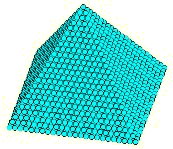May 4, 2016

Categorifying Lucas’ Equation

Posted by John BaezIn 1875, Édouard Lucas challenged his readers to prove this:

A square pyramid of cannon balls contains a square number of cannon balls only when it has 24 cannon balls along its base.

In other words, the 24th square pyramid number is also a perfect square:

$1^2 + 2^2 + \cdots + 24^2 = 70^2$

and this is only true for 24. Nitpickers will note that it’s also true for 0 and 1. However, these are the only three natural numbers $n$ such that $1^2 + 2^2 + \cdots + n^2$ is a perfect square.

This fact was only proved in 1918, with the help of elliptic functions. Since then, more elementary proofs have been found. It may seem like much ado about nothing, but actually this fact about the number 24 underlies the simplest construction of the Leech lattice! So, understanding it better may be worthwhile.

Gavin Wraith has a new challenge, which is to find a bijective proof that the number 24 has this property. But part of this challenge is to give a precise statement of what counts as success!

I’ll let him explain…

He writes:

Do you remember how, seven or so years ago, you kindly posted on the n-Category Café some of my musings about categorifying Nicomachus’s Theorem?

I would like to offer you, and, if you think it suitable, the readers of the n-Category Cafe, another challenge concerning the famed pyramid of 4900 cannonballs (or oranges if you went to the Tate Gallery recently). For I know how you appreciate the rare beauty of the number 24.

Let $S$ (for “square”) be the set of pairs of integers taken from the interval $[1,70]$. Let $P$ (for “pyramid”) be the set of triples $(x,y,z)$ of integers from the interval $[1,24]$ satisfying $x \le z$ and $y \le z$.

The challenge, more aesthetic than mathematical, is to find a “simple” formula for a bijection between $S$ and $P$.

If we write $(M,N)$ for $(24,70)$ then of course we know that

$6 N^2 = M(M+1)(2M+1)$

for which $(1,1)$ and $(24,70)$ are the only positive solutions.

My instincts tell me that the language needed for expressing a bijection $P \to S$ is unlikely to be the simple fragment algebraic+ordering. That is because it needs to encompass the number theory used in Anglin’s short proof, which uses things like quadratic reciprocity:

• W. S. Anglin, The square pyramid puzzle, American Mathematical Monthly 97(2) (Feb. 1990), 120–124.

For my money the predicates “divisible by 2” and “divisible by 3” will play some role.

On a slightly different tack have you come across “super-graphs”, which are to undirected graphs what graded symmetric algebras are to commutative algebras? So in a super-graph (hideous terminology but I use it faute de mieux) there are two kinds of vertex: bosons (degree 0) and fermions (degree 1). There is a binary relationship $\to$ (“edge”) which is antisymmetric between fermions:

$X \to Y \; \iff \; not Y \to X$

and symmetric otherwise. A random countable super-graph has to be isomorphic to the prime numbers with $X \to Y$ meaning X is a square mod Y, where the bosons are primes congruent to 1 mod 4 and the fermions are those congruent to 3 mod 4.

This is just a reformulation of quadratic reciprocity in slightly suggestive language.

Best wishes

Gavin Wraith

Posted at May 4, 2016 6:52 PM UTC

TrackBack URL for this Entry:   https://golem.ph.utexas.edu/cgi-bin/MT-3.0/dxy-tb.fcgi/2879

If $L=[1,4900]$, the map $P \to L$ is given by $(z-1)(z)(2z-1)/6+z(y-1)+x$ represents the lexicographical ordering of triples $(z,y,x)$. So putting in a map $L \to S$, say by providing division with remainder by 70, ends up solving the problem. Which is only that tiny bit further than putting in “divisible by n” predicates, so I feel we need a better measure of simplicity than just the language used.
Of course, this is the most unenlightening solution: we only know that this is a bijection because of Lucas’ result in the first place. That is to say, substitute any other (nontrivial) values of $(M,N)$, and everything will appear okay until you actually try to perform the mapping. It does not and cannot shed any light on the number-theoretical (or numerological) importance of 24.
Perhaps what we’re actually looking for is structures on $S$ and $P$ which make the quadratic reciprocity argument more natural, just like the one Gavin mentions. The difficulty is translating all that modular arithmetic into some map that’s still a bijection.